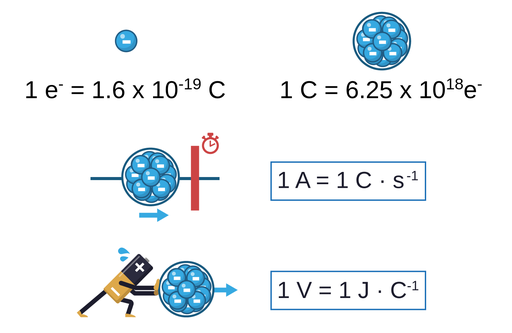In this section, we'll describe what is and what are the main characteristics of Charge, Current and Voltage.

# Charge

The charge is related to the equilibrium between protons and electrons. Electrons carry a negative charge, while protons carry a positive charge. The smallest possible charge is the charge carried by an electron, which is equal to 1.6x10-19 Coulombs (C). 1 Coulomb is then the amount of charge carried by 6.25x1018 electrons. So, to simplify this, we could say that when we talk about 1 Coulomb of charge, it basically means the charge carried by that amount of electrons.

# Current

Current (or current intensity) is the movement of electrically charged particles. Particles like electrons are electrically charged, so when electrons are moving through a conductor (for example a cable) they create an electric current. The unit used to measure current is the Ampere (A). 1 Ampere can be defined as 1 Coulomb per second, which means that at a certain point (of a cable for example) there is 1 Coulomb of charge passing through a certain point of the cable every second.

# Voltage

Voltage can be defined as the difference in electrical potential between two different points. Electrical potential is the equivalent to potential energy for electricity. Basically, the voltage can cause electrically charged particles (i.e. electrons) to move (transform potential energy to kinetic energy), and since the movement of electrically charged particles can be defined as current, voltage can cause a current. Voltage is measured in volts (V). 1 Volt can be defined as 1 Joule per Coulomb. The Joule is the unit used to measure work (work as the physics concept, of course), being work the measurement of the transfer of energy. In this case, a current of 9 volts would be receiving 9 Joules of energy per Coulomb of charge.

When working with circuits, current can be understood as the number of electrons passing through a point in a period of time, while the voltage is the amount of energy provided to those electrons. While voltage (energy) is used by the electrical components of a circuit (light bulb, resistor etc.), the current is not, the electrons do not disappear, the current is constant, since the number of electrons going through the cable is always the same, it's the energy in them (voltage) what changes.Figure 1. Visual representation of charge, current, and voltage.